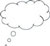# 4 Summary

This unit has encouraged you to think about how to use techniques of visualisation. Visualisation helps students to develop their understanding of operations on fractions such as adding, subtracting, multiplying and dividing by providing ways for them to see, or visualise, what is happening for each operation.

Developing ways to visualise helps students make sense of mathematical notations in many areas – not just fractions. These ideas are important because many students find learning mathematics so traumatic that they simply do not want to think about it. Overcoming this fear will take time and persistence, but making sure that your students are involved in their lessons using the ways such as those described in this unit will help to make sure that your students believe that they can do mathematics.Pause for thought Identify ideas that you have used in this unit that would work when teaching other topics. Make a note now of two topics you have to teach soon where those ideas can be used with some small adjustments.

3 Reading multiplying and dividing fractions differently to help understanding